# EP0158530A2 - Nonrestoring divider - Google Patents

## Info

Publication number
EP0158530A2
EP0158530A2 EP85302455A EP85302455A EP0158530A2 EP 0158530 A2 EP0158530 A2 EP 0158530A2 EP 85302455 A EP85302455 A EP 85302455A EP 85302455 A EP85302455 A EP 85302455A EP 0158530 A2 EP0158530 A2 EP 0158530A2
Authority
EP
European Patent Office
Prior art keywords
partial
output
quotient
predictor
Prior art date
Legal status (The legal status is an assumption and is not a legal conclusion. Google has not performed a legal analysis and makes no representation as to the accuracy of the status listed.)
Granted
Application number
EP85302455A
Other languages
German (de)
French (fr)
Other versions
EP0158530B1 (en
EP0158530A3 (en
Inventor
Masayuki Eshiru Nakahara 404 Ikeda
Current Assignee (The listed assignees may be inaccurate. Google has not performed a legal analysis and makes no representation or warranty as to the accuracy of the list.)
Fujitsu Ltd
Original Assignee
Fujitsu Ltd
Priority date (The priority date is an assumption and is not a legal conclusion. Google has not performed a legal analysis and makes no representation as to the accuracy of the date listed.)
Filing date
Publication date
Priority to JP7035384A priority Critical patent/JPH0340865B2/ja
Priority to JP70353/84 priority
Priority to JP128610/84 priority
Priority to JP59128610A priority patent/JPH0370252B2/ja
Application filed by Fujitsu Ltd filed Critical Fujitsu Ltd
Publication of EP0158530A2 publication Critical patent/EP0158530A2/en
Publication of EP0158530A3 publication Critical patent/EP0158530A3/en
Application granted granted Critical
Publication of EP0158530B1 publication Critical patent/EP0158530B1/en
Anticipated expiration legal-status Critical
Expired - Lifetime legal-status Critical Current

• 241001442055 Vipera berus Species 0.000 claims abstract description 66
• 230000000644 propagated Effects 0.000 claims abstract description 3
• 241000195975 Selaginellaceae Species 0.000 claims 2
• 238000010586 diagrams Methods 0.000 description 15
• 230000000295 complement Effects 0.000 description 10
• 230000000875 corresponding Effects 0.000 description 9
• 230000003247 decreasing Effects 0.000 description 3
• 238000000034 methods Methods 0.000 description 3
• 101710084825 ARG2 Proteins 0.000 description 2
• 101710005038 CPA1 Proteins 0.000 description 2
• 101710084186 CPA2 Proteins 0.000 description 2
• 102100003771 Carboxypeptidase A2 Human genes 0.000 description 2
• 102000032843 Monovalent Cation:Proton Antiporter-1 Human genes 0.000 description 2
• 238000010276 construction Methods 0.000 description 2
• 101710070568 CSA1 Proteins 0.000 description 1
• 101710008504 RMD6 Proteins 0.000 description 1
• 230000000052 comparative effects Effects 0.000 description 1
• 239000000203 mixtures Substances 0.000 description 1
• 230000001902 propagating Effects 0.000 description 1

## Images

•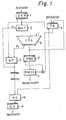•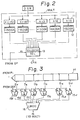•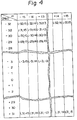••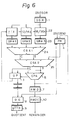•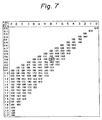•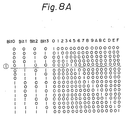•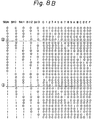•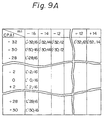•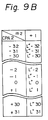## Classifications

• GPHYSICS
• G06COMPUTING; CALCULATING; COUNTING
• G06FELECTRIC DIGITAL DATA PROCESSING
• G06F7/00Methods or arrangements for processing data by operating upon the order or content of the data handled
• G06F7/38Methods or arrangements for performing computations using exclusively denominational number representation, e.g. using binary, ternary, decimal representation
• G06F7/48Methods or arrangements for performing computations using exclusively denominational number representation, e.g. using binary, ternary, decimal representation using non-contact-making devices, e.g. tube, solid state device; using unspecified devices
• G06F7/52Multiplying; Dividing
• G06F7/535Dividing only
• GPHYSICS
• G06COMPUTING; CALCULATING; COUNTING
• G06FELECTRIC DIGITAL DATA PROCESSING
• G06F7/00Methods or arrangements for processing data by operating upon the order or content of the data handled
• G06F7/38Methods or arrangements for performing computations using exclusively denominational number representation, e.g. using binary, ternary, decimal representation
• G06F7/48Methods or arrangements for performing computations using exclusively denominational number representation, e.g. using binary, ternary, decimal representation using non-contact-making devices, e.g. tube, solid state device; using unspecified devices
• G06F7/49Computations with a radix, other than binary, 8, 16 or decimal, e.g. ternary, negative or imaginary radices, mixed radix non-linear PCM
• GPHYSICS
• G06COMPUTING; CALCULATING; COUNTING
• G06FELECTRIC DIGITAL DATA PROCESSING
• G06F7/00Methods or arrangements for processing data by operating upon the order or content of the data handled
• G06F7/38Methods or arrangements for performing computations using exclusively denominational number representation, e.g. using binary, ternary, decimal representation
• G06F7/48Methods or arrangements for performing computations using exclusively denominational number representation, e.g. using binary, ternary, decimal representation using non-contact-making devices, e.g. tube, solid state device; using unspecified devices
• G06F7/52Multiplying; Dividing
• G06F7/535Dividing only
• G06F7/537Reduction of the number of iteration steps or stages, e.g. using the Sweeny-Robertson-Tocher [SRT] algorithm
• G06F7/5375Non restoring calculation, where each digit is either negative, zero or positive, e.g. SRT

## Abstract

A divider apparatus includes a divisor register (DSR) for setting a divisor, a partial remainder register (PR) for setting a dividend or a partial remainder, a predictor (QP) for predicting a partial quotient, a multiplier (MULT) for multiplying the content of the divisor register, and a first adder (ADDER 2) for subtracting the output of the multiplier from the content of the partial remainder register and for calculating the partial remainder. The apparatus further inludes a second adder (ADDER 1) for determining the difference between the upper digits of the multiplier and the upper digits of the partial remainder register, a first predictor (QP2) for predicting the partial quotient from the output of the first adder and the upper digits of the divisor register, a second predictor (QP1) for predicting the partial quotient in the third cycle from a corrected output of the second adder and the digits of the divisor register, a first element (CLA) for determining the carry to be propagated to the second adder from remainder digits of the multiplier and remainder digits of the partial remainder register, and a second element (SEL) for selecting either the output of the first predictor-or the output of the second predictor by using the output of the first element, so that the output of the second element corresponds to a predicted quotient.

## Description

NONRESTORING DIVIDER
• This invention relates to a nonrestoring divider and, more particularly, to a high speed circuit construction by which a circuit for predicting a partial quotient is formed using a smaller amount of hardware.
• Conventionally, a momrestoring divider is used as one element of a divider system. In such a system, sets of quotients used for forming digits of the quotient are controlled in such a manner that where sets of quotients exist which do not include a zero, then each digit of the quotient is selected therefrom.
• These particular sets of the quotients having no zero are usually expressed as follows, wherein "r" represents the radix.
• -(r - 1), -(r - 2),..., -1, +1, ..., r - 2, r - 1 In many calculators, calculation is carried out by using a plurality of bit units rather than just one bit unit and, obviously, a radix larger than 2 is used.
• For example, the radix is 4 in a 2 bit unit, and becomes 8 in a 3 bit unit.
• Usually, an operation unit of ℓ bits is an m digit figure having r as the radix, and is expressed as; r = 2ℓ/m
• A characteristic feature of nonrestoring division is the use of a negative number in the digits as a result of the operation by remaining the inversion of negative and the positive of the dividend formed when the digit of the result of the operation is determined, and the dividend or multiple of the dividend is added or subtracted by the sign for the dividend by using the negative number in the result of the operation.
• For example, the values multiplying the dividend by k [i.e., the multiple -(= - 1), -(r - 2), ..., -1, +1, ..., r - 2, r - 1] are set in the divisor registers, the divisor registers are selected by a predicting signal output from a partial quotient predictor, and the quotient is obtained by repeatedly adding and subtracting the values multiplying the dividend by k.
• In such a nonrestoring divider system, when the number of bits "n" used as the operation unit becomes large, the radix is increased, for example to 2n, so that the number of operation repetitions can be decreased and high speed operation can be expected. However, the multiplying of the divisor becomes complex, and therefore the predicting logic for the quotient must be precise, which gives rise to the problem that the number of circuits must be considerably increased.
• Concerning the logic for predicting the partial quotient, a method has not been developed for forming an effective predicting circuit for the partial quotient.
• An object of the present invention is to provide a nonrestoring divider which can decrease the time needed for predicting the partial quotient.
• Another object of the present invention is to provide a nonrestoring divider which can decrease the amount of hardware used for the logic needed for predicting the partial quotient.
• According to the invention there is provided a divider apparatus which includes a divisor register for setting a divisor; a partial remainder register for setting a dividend or a partial remainder; a predictor for predicting a partial quotient; a multiplier for multiplying the content of the divisor register; a first adder for subtracting the output of the multiplier from the content of the partial remainder register and for calculating the partial remainder, so that the predictor predicts the partial quotient from upper digits of the divisor register and upper digits of the partial remainder, and also repeats a cycle which sets the output of the adder at the partial remainder register so as to carry out the division, characterised by a second adder for determining the difference between the upper digits of the multiplier and the upper digits of the partial remainder register, a first predictor for predicting the partial quotient from the output of the second adder and the upper digits of the divisor register; a second predictor for predicting the partial quotient from a corrected output of the second adder and the upper digits of the divisor register; first means for determining a carry to be propagated to the second adder from the remainder digits of the multiplier and the remainder digits of the partial remainder register; and second means for selecting either the output of the first predictor or the output of the second predictor by using the output of the first means, thereby making the output of the second means correspond to a predicted quotient.
• Embodiments of the invention will now be described, by way of example, with reference to the accompanying drawings, in which
• Figure 1 is a diagram showing the principle of one example of a conventional nonrestoring divider apparatus;
• Figure 2 is a diagram showing the principle of one example of a multiplier shown in Figure 1;
• Figure 3 is a diagram showing the principle of one example of a circuit for predicting a partial quotient shown in Figure 1;
• Figure 4 is a diagram showing the content of the circuit for predicting a partial quotient;
• Figure 5 is a block diagram of one embodiment of a nonrestoring divider apparatus according to the present invention;
• Figure 6 is a diagram showing an improvement of the mutiplier shown in Figure 5;
• Figure 7 is a diagram showing the content of the circuit for predicting a partial quotient shown in Figure 5;
• Figures 8A and 8B are diagrams showing the logic states of the decoder shown in Figure 5; and
• Figures 9A and 9B are diagrams showing another embodiment of the content shown in Figure 7.
• Figure 1 is a block diagram of one example of a conventional restoring divider comprising a divisor register (DSR) 1 in which a divisor is stored and the divisor is fed to a multiplier (MULT) 2. The multiplier 2 receives a partial quotient predicting signal (hereinafter shown as "m") from a partial quotient predictor (QP) 3 and forms dividends multiplied by -15, -14, -13, ..., -2, -1, 0,. +1, +2, ..., +14, +15 when the radix is 16. Note, in this circuit a method in which whole multiples are previously formed and selected, a method which uses a general adder, or a method which calculates by using a smaller number of divisor registers than the radix and multi-stage carry/save adders can be used.
• In a partial remainder register (PR) 4, after the dividend is set at the first operation cycle, a new partial remainder is set at every operation cycle. A carry propagate adder (CPA) 5 carries out the addition of -the partial remainder register 4 and a divisor multiplied by m (-15 = m ≦ +15: where m is an integer), and the result thereof is output to the partial quotient register 4, the partial quotient predictor 3, and a remainder register 6.
• The remainder register 6 holds the final predicted remainder for the repetition operation, and after the adding and subtracting operations are repeated, a correct quotient is output via a remainder corrector (RMDC) 7. In a concrete correcting method in the remainder corrector (RMDC) 7, when a sign bit of the remainder register (RMD) 6 is negative, the complement of 2 is determined as the quotient, and when the sign bit of the remainder register 6 is positive, the value is determined as the quotient.
• A partial quotient generator (QG) 8 compares the output of the partial quotient predicting circuit (QP) 3 and the sign bit of the partial remainder register (PR) 4, so that a correct partial quotient is determined and stored in a quotient register (QR) 9.
• The partial quotient predicting circuit (QP) 3, according to the present invention, calculates the value of m in m x DSR to be calculated next from the output of the carry propagate adder (CPA) 5 (hereinafter referred to as CPA) and the output of the divisor register (DSR) 1 (hereinafter referred to as DSR). Logically, the circuit (QP) 3 uses the entries in the above-mentioned CPA and DSR to retrieve the table for m.
• However, when the CPA and DSR are used in this way, the table is extremely large. For example, in the nonrestoring dividing operation having a radix of 16, including the sign bit, the following table will be formed:
• CPA: 6 bits (64 entries)
• DSR: 9 bits (256 entries)
wherein, as shown hereinafter, it is normalized so that the most significant bit becomes 1.
• Therefore, in practice, the CPA and m are used as the entry, and the DSR table is formed, in such a manner that the table is reversely retrieved. In the table mentioned above, m becomes 5 bits including the sign, CPA becomes 6 bits including the sign, and as mentioned above, the size of the table becomes 64 x 32 entries, so that the size of the table is decreased to about 1/8. Referring to the adding and subtracting result (CPA) = 0,. the table above is almost symmetrical, and the possibility of retrenchment is included.
• Figure 2 is a diagram showing the principle of the multiplier shown in Fig. 1. In Fig. 2, 11 designates multiply circuits [x(-15) ~ xl5], 12 designates divisor registers (-15DSR ~ +15DSR), and 13 designates a selector. In the conventional system, when the radix is 16, thirty kinds of multiplier registers -15x divisor, -14x divisor, ... , -2x divisor, -lx divisor, 2x divisor, ... , 14x divisor, 15x divisor are necessary. These thirty kinds of data are formed by the multiply circuits [x(-15), ..., x15] 11, the outputs are held in the divisor registers (-15DSR ~ +15DSR) 12, and the outputs of the divisor registers 12 are supplied to the selectors 13. In the selector 13, the values held in the registers 12 are selected by the signal m, that is, one of the multiple thirty kinds of data is selected.
• Figure 3 is a diagram showing a principle of the partial quotient predicting circuit shown in Fig. 1. In Fig. 3, the six upper bits of the carry propagating adder 5 are supplied to a memory 14 as an address, and in the memory 14, the contents are divided into thirty one groups each having 9 bits, that is DF, DE, ..., DO, D-1, D-2 ... D-F. The divided values DF, DE, ... , DO, D-1, ... , D-F are supplied to comparators 15F ... 15O, ... 15-F. Further, the upper five bits of the divisor (DSR) is supplied to other input terminals of the comparators 15F ~ 15O. In the comparators 15F ~ 15O, the input is formed by a 9 bit signal, the output thereof is a one bit signal. When the inputs have the relationship A > B, the output becomes "I", and when the inputs have the relationship A < B, the output becomes "0". In Fig. 3, 16 is a circuit for detecting "1" bit in the left most side.
• Figure 4 shows a precise table which receives the CPA and m as entries and designates the DSR. The table shown in Fig. 4 has 64 x 31 entries. In Fig. 4, CPAO shows the value at which the upper 6 bits (including the sign) of CPA output are extracted and designates the decimal number, and m is the signal of the partial quotient predicting circuit (QP) 3 designated by the decimal number.
• Such table look up system can be replaced by a random logic circuit which performs the same function.
• The CPAO row m column in the partial quotient predicting table [QPT(CPAO, m)] designates the logical function whereby the upper 9 bits of the DSR (hereinafter referred to as DSRO) are input, and these have the value "1" or "0", (hereinafter, referred to as [LcpaO, m(DSRO)]).
• For the purpose of simplifying the explanation, it is assumed that the DSR is normalized so that it is a positive integer and the most significant bit becomes 1.
• First, the principle of the nonrestoring divider will be explained. When the divisor is D, the dividend is Pn, the partial remainder is Pn, and the partial remainder predicting signal is m; then the dividing operation in the nonrestoring divider is expressed by the following asymptotic equation, and in the n+l'th quotient Qn+1, it is sufficient that the partial quotient satisfies the following condition, Therefore, the logic function "LcpaO, m(DSRO)" has the following conditions.
• and this function becomes 0, except for the above,
• ② When the following relationship exists between m and m' wherein one is determined as "1" and the other is determined as "0".
• ③ The condition wherein the partial quotient predicting table formed by using the process mentioned above functions is that where one m exists for all CPAO, DSRO, and the following equation is satisfied for such an m This condition may be said to be "CONDITION I".
• The partial quotient predicting table formed by above-mentioned method is shown in Fig. 4. In Fig. 4, when a DSRO having 9 bits is input, only one m exists for all CPAO (64 bits) and the corresponding logic function is
• If the DSRO is 8 bits, only one m exists for all CPAO and the condition: is not satisfied, so that the table does not function as the partial quotient predicting table.
• Further, if the DSRO is 10 bits, it becomes redundant since only one m exists for all CPAO. Therefore, when the DSRO is 9 bits, this may be said to be the most suitable number of bits in the DSRO for retrieving m in the nonrestoring divider wherein the radix is 16.
• Figure 5 is a block diagram of one embodiment of the nonrestcring divider apparatus according to the present invention. In Fig. 5, the same symbols correspond to the same elements as shown in Fig. 1.
• In Fig. 5, an adder 51 adds the upper bits of the partial remainder register (PR) 4 and the upper bits of output of the multiplier (MULT) 2 that is, the upper bits of mDSR without using the carry signal from an adder 52 (the output signal of the carry predicting circuit (CLA) 521), and the output thereof is supplied to a complement generator 10 and the remainder register (RMD) 6.
• In the complement generator 10, when the sign bit of the adder (ADDER 1) 51 is "1", the complement of the input data (output of the adder 51) is output to the decoder 11, and when the sign bit is "0", the input data is directly output to the decoder 11.
• In the -decoder 11, the result obtained from the complement generator (COMP) 10 is decoded and supplied to the partial quotient predicting circuit (QP2) 3.
• An adder (ADDER 2) 52 receives the lower bits of the partial remainder register (PR) 4 and the lower bits of the output (mDSR) of the multiplier (MULT) 2, and a carry look-ahead circuit (CLA) 521 is attached thereto for the purpose of carrying out high speed operations.
• The output of the adder 51 is also input to a carry adding circuit 53 and compensated by the output of CLA 521 and fed to RMD6 by being concatinated with the output of adder 52.
• The characteristic feature of the present invention is to provide new functional blocks, that is, a modified decoder circuit (MDEC) 111, a selector (SEL) 12, and a partial quotient predicting circuit (QPl) 31 having the same function as that of the partial quotient predicting circuit (QP) 3 explained in Fig. 1 to Fig. 4.
• The basic operation of the apparatus shown in Fig. 5 is similar to the conventional system, therefore, only the characteristic feature of the present invention will be explained hereinafter.
• First, the modified decoder circuit (MDEC) 111 forms, in principle, the decode signal assuming +1 when the sign bit of the adder (ADDER 1) 51 is "0" (positive) and the decode signal assuming -1 when the sign bit of the adder 51 is "1" (negative). The selector 12 selects either the partial quotient predicting circuit (QP1) 3 or (QP2) 31 by using the output signal of the carry predicting circuit (CLA) 521.
• Concretely, when the output of the carry predicting circuit (CLA) 521 is "1" (that is, a carry exists), the selector 12 selects the partial quotient circuit (QP1) 31, and where the output of the circuit (CLA) 521 is "0" (that is, no carry exists), the partial quotient circuit (QP2) 3 is selected. The function of the modified decoder circuit (MDEC) will be explained in detail later referring to Figs. 8A and 8B.
• Figure 6 is a block diagram of another embodiment of the present invention.
• In Fig. 6, 22 designates multipliers (±1x, +2/+4x, +8/+16x) which are formed by a complement circuit and a shifter, wherein multiplication in the + side is achieved by shift only and multiplication in the - side is achieved by using the complement; 23 designates divisor registers (SR #1, SR #2, SR #3); 24 and 25 carry save adders (CSAl, CSA2) having three inputs; C designates a carry signal; and S designates a sum signal.
• In the circuit shown in Fig. 6, the divisor registers 23 [corresponding to (+15DSR ~ -15 DSR) in Fig. 2] are formed by three registers (SR #1 ~ SR #3) the number of which is smaller than the number of bits (4), and the outputs of four registers SR #1, SR #2, SR #3 and the partial remainder register(PR) 4 are added by using two carry/save adders 24, 25 having three inputs.
• When the divisor is set in the divisor register (DSR) 1 and the dividend is set in the partial remainder register (PR) 4, the dividend is supplied via the three-input carry/save adder (CSA 2) 25 to the partial quotient predicting circuit (QP) 3, and a signal predicting the most significant digit of the quotient is output. This predicting signal selects a plurality of routes by which the output of the divisor register 1 is multiplied by the adders 22 (+lx, +2/+4x, +8/+16x), and the outputs thereof are set in the divisor registers (SR #1, SR #2, SR #3) 23.
• Next, the outputs of the divisor registers (SR #1, SR #2, SR #3) 23 and the output of the partial remainder register (PR) 4 are added by using the three-input carry/save adders (CSA1, CSA2) 24, 25 and the carry propagation adder 5, and the output thereof is again input to the partial remainder register (PR) 4.
• The output (C, S) of the three-input carry/save adder (CSA2) 25 is supplied to the partial quotient predicting circuit (QP) 3, so as to determine the inputs of the three kinds of divisor registers (SR #1, SR #2, SR #3) which will be selected next.
• Fig. 7 is a diagram showing one embodiment of the partial quotient predicting table according to the present invention.
• First, the concept of the present invention will be explained. At the i'th step of the non-restoring division, the following equations are used. wherein pi designates a partial remainder, d is a divisor, r is a radix, and when the radix is r, mi is expressed as
• The value mi is selected so that the value of Pi+1 obtained by calculating the equation ① satisfies the equation ②, and the equation ① is calculated by using the selected m..
• In the actual computer, at the best case, the upper bits of pi and d [corresponded to Pi in CPA and D in DSR] are extracted by digits sufficient to ensure accuracy in determining mi, the value of mi is - determined by using the partial quotient predicting table, and thereafter precise Pi+1 is calculated by using equation ①.
• As an operation for mi x d, a method for using the multiplier, a method for using a divisor register at every multiple, a method for using carry/save adders, etc., are well known and the subtraction pi - mi x d is carried out by using a well known adding and subtracting circuit, etc.
• The feature of the present invention lies within the carrying out of the function of determining mi which satisfies the equation ② from Pi and D above by using the smallest possible amount of hardware.
• As mentioned above, when P. , D are fixed, a plurality values of mi exist which satisfy the equations ①, ② at the same time.
• When the quotient sets in the nonrestoring divider is x [= (-r, -r + 1, ... , -1, 0, 1, ... , r - 1 , r) wherein r is a radix], the set M(Pi, D) of mi, which satisfies the equations ①, ② at the i'th step, is a partial set of X, and usually a plurality of elements exist. That is,
• In other words, when Pi ≧ 0, a suitable mi is determined by selecting one element from M'(Pi , D), and when Pi < 0, a suitable mi is determined by selecting one element from N[M'(Pi, D)].
• Therefore, when the system of the present invention is used, the table which determines mi from any Pi and D is provided only for Pi ≧ 0, so that the size of the table can be reduced by about one-half.
• The above-mentioned will be explained by using the example of the nonrestoring division by which the quotient having 4 bits (that is radix r = 16) is obtained in one cycle time (one step).
• When r = 16, for the purpose of determining m. , Pi requires 6 bits including the sign and D requires 9 bits (however, for the purpose of simplicity, it is previously normalized so that D is positive and the most significant bit is "1").
• Further, the following relationship exists between the accuracies of the P. , D mentioned above. That is, when one is rough, the other requires high accuracy. The above relationship, that is, Pi = 6 bits and D = 9 bits, is one combination relating to that relationship.
• Accordingly, in accordance with the definition of the mapping, the following relationship can be obtained: Also, when Pi < 0, the same result as that calculated above is obtained by the table of M'(Pi, D). Therefore, it is known that the correct result can be obtained even if such a table is used.
• Thus, the elements of the partial sets M(Pi, D), M(P i, D) can be obtained by providing one table of M'(Pi, D).
• Figure 7 shows the table in which the relationship between Pi, D, and mi is obtained by using the above-mentioned principle.
• In Fig. 7, mi is 4 bits, Pi is 5 bits without a sign, D is 9 bits (however, it is normalized so that the most significant bit becomes "1"), and several discontinuous points exist therebetween. However, it is known that a comparative continuous relationship exists, that is, when D increases, mi decreases.
• As mentioned above, the process for determining mi logically corresponds to the retrieval of the table which uses P. and D as entries to determine the value of mi. As Pi becomes 6 bits including the sign, a table must be formed having 64 entries x 256 entries, and this is not practical.
• Therefore, it is considered that the construction of the table which uses Pi, mi as entries and the range for D as elements as shown in Fig. 6 is the most efficient.
• In the table shown in Fig. 7, the upper 5 bits of Pi are designated as a hexadecimal number, the 4 bits of mi are designated as a hexadecimal number, and the upper 9 bits (wherein the most significant bit is "1") of D are designated as a hexadecimal number. The upper 9 bits of D designates a lower limit value for the elements entry.
• As a concrete example, when Pi = '001011' is designated as a hexadecimal number without a sign, it becomes 'OB'(H), and similarly, D = '110000000' becomes '180'(H). Therefore, in the table, as and referring to the following relationship
• it is known that the value to be determined is '8'. When the following relationship is given the sign is negative, so that '001011' is obtained as the complement of (Pi)b. Therefore, 0B' excepting the sign can be designated as a hexadecimal number, and the entry is the same as mentioned above, and thus mi = 8 is obtained. Finally, the sign is adjusted, and '-8' is obtained as a true predicting partial quotient.
• Fig. 8A shows one example of the function of the usual decoder (DEC) 11 shown in Fig. 5 and the function of the modified decoder (MDEC) 111 shown in Fig. 5.
• The example of the operation of the invention by which the partial quotient predicting signal m is output when the output data of the adder 51 [that is, the upper digit of PRi + mDSR] is positive and negative.
• [Example 1]
• When the output data of the adder 51 is '00100' the sign bit of the data is "0" (positive). Therefore a carry is not input from the carry predicting circuit 521, and the output of the decoder 11 [that is the decoded output which makes the line 4 (see Fig. 8A) to an ON state, shown by ① in Fig. 8A] is supplied to the partial quotient predicting circuit 3. When the obtained partial quotient predicting signal has a carry, it is predicted that the above-mentioned data will become '00101' and the sector 12 exerts a control through the carry from the carry predicting circuit 52 so that the output of the decode circuit input as '00100' including the sign [shown in ② in Fig. 8B, that is, the output which places the line 5 (see Fig. 8B) to an ON state] is supplied to the modified decoder 111, and the input partial quotient predicting signal is supplied to the partial quotient predicting circuit 31. Thus, the correct partial quotient predicting signal m is obtained.
• [Example 2]
• When the output data of the adder 51 is '11011', the sign bit of the data is "1" (negative).. Therefore, according to the logic disclosed in the prior Japanese Unexamined Application No. 59-070353, the output of the adder 51, that is the complemental output of "1", is supplied to the decoder circuits 11 and 111, and the absolute value of the partial quotient predicting. signal m is output.
• Therefore, in this case, if no carry from the carry predicting circuit 521 exists, the complement of 1 of the data '1011' excepting the sign is '0100'. Then the output [shown in ① on Fig. 8A, that is, the decoded output which places the line 4 to an ON state] of the decoder 11 corresponding to the complemental output is supplied to the partial quotient predicting circuit 3. When the output of the partial quotient predicting circuit 3 includes a carry, the original data becomes '11100' and then it is predicted that the complemental output will become '0011'. Then the selector 12 exerts control through the carry from the carry predicting circuit 521 so that the output of the decode circuit input as '10100' including the sign [shown in ③ on Fig. 7B, that is, the output which places the line 3 to an ON state] is supplied to the modified decoder 111 and the input partial quotient predicting signal is supplied to the partial quotient predicting circuit 31. Thus, the correct partial quotient predicting signal m is obtained.
• As mentioned above, the characteristic feature is that, the modified decoder 111 forms the decode signal predicting +1 when the sign bit of the adder 51 is principally "0" positive, and forms the decode signal predicting -1 when the sign bit thereof is "1" (negative).
• Figures 9A and 9B are an alternative to the example shown in Fig. 7.
• In the table shown in Fig. 4, the CPAO is 6 bits (64 entries), the obtained-m is 5 bits including the sign (32 entries), and the radix is 16. However, also when m is 4 bits including the signal (expressed by ml), δ = (1/2)5 [that is, the table is formed by inputting the upper 6 bits (DSRl) of the DSR] and ε = 2 [the CPA is 4 bits (CPAl) including the sign], the table which satisfies the table shown in Fig. 4, (that is, the CONDITION I above) can be obtained.
• This table is a rough portion of quotient predicting table shown in Fig. 9A.
• When the table is as shown in Fig. 9A and as shown in Fig. 4, the following can be known. That is, even when the portion corresponding to add number m in the precise portion of the partial quotient predicting table shown in Fig. 4 is "1", the value ml smaller by "1" than in the table shown in Fig. 4 is obtained in the table shown in Fig. 9A.
• Therefore, for the purpose of correcting the error in both cases, when "1" exists in the portion for the odd number m in the table shown in Fig. 4, the precise m can be obtained by storing its information as another correcting table and by referring to the same. Figure 9B is a table which schematically shows the above mentioned correcting table.
• Hereinafter, the correcting method will be concretely explained.
• First, referring one column CPA1 = -30 in the rough portion of the quotient predicting table, when, for example,
• When the corrected value in the same column is this shows that "1" does not exist at any portion for the odd number m for CPAO = -30. Therefore, in the rough portion of quotient predicting table, for example, is used as it stands as the precise portion of quotient predicting table.
• Further, when in one column in CPA1 = -30 in the rough portion of the quotient predicting table, for example, and in the column in CPA2 = -29 in the correcting table, the correction is carried out to the value corresponding to the odd number m (i.e., corresponding to m = -13), that is
• That is, when L" -30 = 1 in the correcting table, it shows that, in the column corresponding to CPAO = -30 in the precise portion of quotient predicting table shown in Fig. 4, one "1" exists at any portion for the odd number m. This is retrieved from the rough portion of quotient predicting table shown in Fig. 9A, and when as mentioned above, it is determined that
• This is the gist of the present invention.
• The above-mentioned correcting table is formed by the above-mentioned condition, and each element thereof is expressed by the following equation.
• L"cpa2, m2(DSR2) = ELcpa2, 2i+l(DSR2) = 1 wherein I shows the logical sum from i = -8 to i = +7, cpa2 is the upper 6 bits including the sign in the output of the carry propagate adder, and DSR2 is the upper 9 bits of the dividend.
• The correcting table formed by the equation showing the condition mentioned above is shown in Fig. 9B.
• According to the present invention, the same function as shown in the precise portion the quotient predicting table shown in Fig. 4 can be realized by the rough portion of the quotient predicting table shown in Fig. 9A and the correcting table shown in Fig. 9B, so that it is recognized that the amount of hardware needed can be decreased.
• In the explanation above, CPA and m are used as the entry of the partial quotient predicting table, however, m can be placed to the value coded by using a plurality of desired values from -16 to +15, to form an effective signal in the subsequent process.

## Claims (6)

2. Apparatus according to claim 1, characterised in that the first predictor (QP1)/second predictor (QP2) output positive partial quotient (m) when the output of the second adder (ADDER 1) is positive, and a negative partial quotient (m) when the output of the second adder is negative, and feed the quotient correcting circuit (QG) which corrects the predicted m by using the sign of the partial remainder formed by the partial quotient so as to determine a true value of the quotient.
3. Apparatus according to claim 2, characterised in that the first predictor (QP1)/second predictor (QP2) respectively comprise a circuit (COMP) by which when the output of the second adder (ADDER 1) is + (or -), the value is not changed and when the output of the second adder is - (or +), the complemental value is determined; a circuit for determining the partial quotient (m) from the output of the complemental value determining circuit (COMP) and the upper digits of the divisor register (DSR); and a circuit for compensating the partial quotient (m) by the sign of the output of the second adder.
4. Apparatus according to claim 3, characterised in that the circuit for determining the partial quotient further comprises means for outputting partial bits of the partial quotient by using partial bits of the output of the complemental value determining circuit (COMP) and means for outputting remainder bits of the partial quotient by using whole bits of the output of said determining circuit (COMP).
5. Apparatus according to claim 2, characterised in that the first predictor (QP1)/ second predictor (QP2) comprise means for outputting partial bits of the partial quotient by using partial bits of the output of the second adder (ADDER 1) and means for outputting remainder bits of the partial quotient by using whole bits of the output of the second adder.
6. Apparatus according to any one of claims 1 to 5, characterised in that the multiplier comprises a shift circuit which generates the values multiplying the value of the divisor register by ±1, ±2, ±4, ...±2i; means for selecting the combination of the multiples in accordance with the value of the partial quotient; and an adder for adding the selected multiples.
EP85302455A 1984-04-09 1985-04-04 Nonrestoring divider Expired - Lifetime EP0158530B1 (en)

## Priority Applications (4)

Application Number Priority Date Filing Date Title
JP7035384A JPH0340865B2 (en) 1984-04-09 1984-04-09
JP70353/84 1984-04-09
JP128610/84 1984-06-22
JP59128610A JPH0370252B2 (en) 1984-06-22 1984-06-22

## Publications (3)

Publication Number Publication Date
EP0158530A2 true EP0158530A2 (en) 1985-10-16
EP0158530A3 EP0158530A3 (en) 1987-12-09
EP0158530B1 EP0158530B1 (en) 1992-02-05

# Family

## Family Applications (1)

Application Number Title Priority Date Filing Date
EP85302455A Expired - Lifetime EP0158530B1 (en) 1984-04-09 1985-04-04 Nonrestoring divider

## Country Status (8)

US (1) US4722069A (en)
EP (1) EP0158530B1 (en)
KR (1) KR890003321B1 (en)
AU (1) AU553078B2 (en)
BR (1) BR8501629A (en)
CA (1) CA1231455A (en)
DE (1) DE3585334D1 (en)
ES (1) ES8609768A1 (en)

## Cited By (7)

* Cited by examiner, † Cited by third party
Publication number Priority date Publication date Assignee Title
EP0416153A1 (en) * 1989-09-07 1991-03-13 Siemens Nixdorf Informationssysteme Aktiengesellschaft Method for dividing any-length operands respectively normalized at the beginning for data processing equipment and digital divider for carrying out the method
GB2239536A (en) * 1989-11-15 1991-07-03 United Technologies Corp Binary division of signed operands
EP0436905A2 (en) * 1990-01-09 1991-07-17 Digital Equipment Corporation High performance adder using carry predictions
EP0452099A2 (en) * 1990-04-10 1991-10-16 Matsushita Electric Industrial Co., Ltd. Divider unit
DE4121106A1 (en) * 1991-06-26 1993-01-07 Siemens Nixdorf Inf Syst Radix division of binary numbers in data processing system - subjecting divisor to reduction process followed by comparison process to generate part quotients
EP0529101A1 (en) * 1991-03-13 1993-03-03 Fujitsu Limited Floating-point dividing circuit
EP0562137A1 (en) * 1992-03-24 1993-09-29 Siemens Nixdorf Informationssysteme Aktiengesellschaft Method for dividing normalized operands

## Families Citing this family (22)

* Cited by examiner, † Cited by third party
Publication number Priority date Publication date Assignee Title
US4939686A (en) * 1987-05-18 1990-07-03 Weitek Corporation Method and apparatus for shared radix 4 division and radix 4 square root
JPH0786826B2 (en) * 1988-07-19 1995-09-20 日本電気株式会社 Integer division circuit
US5132925A (en) * 1988-08-18 1992-07-21 Digital Equipment Corporation Radix-16 divider using overlapped quotient bit selection and concurrent quotient rounding and correction
US5023827A (en) * 1988-08-18 1991-06-11 Digital Equipment Corporation Radix-16 divider using overlapped quotient bit selection and concurrent quotient rounding and correction
US4992968A (en) * 1989-02-17 1991-02-12 Digital Equipment Corporation Division method and apparatus including use of a Z--Z plot to select acceptable quotient bits
US4996660A (en) * 1989-04-17 1991-02-26 International Business Machines Corporation Selection of divisor multipliers in a floating point divide circuit
US5103420A (en) * 1989-12-04 1992-04-07 Dror Avnon Method and apparatus for srt division using gray coded quotient bit signals
US5128891A (en) * 1990-04-02 1992-07-07 Advanced Micro Devices, Inc. High speed divider with square root capability
KR920006322B1 (en) * 1990-04-03 1992-08-03 정호선 Divider
KR920009092B1 (en) * 1990-04-03 1992-10-13 정호선 Divider circuit
US5031138A (en) * 1990-06-04 1991-07-09 International Business Machines Corporation Improved ratio decoder for use in a non-restoring binary division circuit
JP2835153B2 (en) * 1990-06-25 1998-12-14 株式会社東芝 High radix divider
JPH04172526A (en) * 1990-11-07 1992-06-19 Toshiba Corp Floating point divider
JPH0731592B2 (en) * 1990-11-29 1995-04-10 株式会社東芝 Division circuit
JP2502836B2 (en) * 1991-03-19 1996-05-29 富士通株式会社 Preprocessing device for division circuit
EP0543024B1 (en) * 1991-06-07 1998-09-02 Fujitsu Limited Divider
US5381380A (en) * 1992-05-18 1995-01-10 Sharp Kabushiki Kaisha Divide circuit having high-speed operating capability
US5239498A (en) * 1992-08-31 1993-08-24 Intel Corporation Methods and apparatus for improved quotient correction in nonrestoring division computation circuits
US6108723A (en) * 1998-07-20 2000-08-22 Hewlett-Packard Company System for implementing hardware automated control of burst mode data transfer over a communication link between devices operating in a block mode
US7149767B2 (en) * 2003-05-12 2006-12-12 International Business Machines Corporation Method and system for determining quotient digits for decimal division in a superscaler processor
US8140608B1 (en) * 2007-05-31 2012-03-20 Nvidia Corporation Pipelined integer division using floating-point reciprocal
JP5169760B2 (en) * 2008-01-28 2013-03-27 富士通株式会社 Communication device, reception data size check method, multiple determination circuit, and multiple determination method

## Citations (3)

* Cited by examiner, † Cited by third party
Publication number Priority date Publication date Assignee Title
US3852581A (en) * 1972-12-14 1974-12-03 Burroughs Corp Two bit binary divider
JPS56123038A (en) * 1980-03-04 1981-09-26 Fujitsu Ltd Division control system
JPS58142441A (en) * 1982-02-18 1983-08-24 Toshiba Corp High radix division system

## Family Cites Families (1)

* Cited by examiner, † Cited by third party
Publication number Priority date Publication date Assignee Title
US4320464A (en) * 1980-05-05 1982-03-16 Control Data Corporation Binary divider with carry-save adders

## Patent Citations (3)

* Cited by examiner, † Cited by third party
Publication number Priority date Publication date Assignee Title
US3852581A (en) * 1972-12-14 1974-12-03 Burroughs Corp Two bit binary divider
JPS56123038A (en) * 1980-03-04 1981-09-26 Fujitsu Ltd Division control system
JPS58142441A (en) * 1982-02-18 1983-08-24 Toshiba Corp High radix division system

## Non-Patent Citations (3)

* Cited by examiner, † Cited by third party
Title
IBM TECHNICAL DISCLOSURE BULLETIN, vol. 24, no. 11B, April 1982, pages 5812-5819, New York, US; K.G. TAN: "Transformed high-radix division for high performance computers" *
PATENT ABSTRACTS OF JAPAN, vol. 5, no. 201 (P-94), 19th December 1981; & JP-A-56 123 038 (FUJITSU K.K.) 26-09-1981 *
PATENT ABSTRACTS OF JAPAN, vol. 7, no. 260 (P-237), 18th November 1983; & JP-A-58 142 441 (TOKYO SHIBAURA DENKI K.K.) 24-08-1983 *

## Cited By (12)

* Cited by examiner, † Cited by third party
Publication number Priority date Publication date Assignee Title
EP0416153A1 (en) * 1989-09-07 1991-03-13 Siemens Nixdorf Informationssysteme Aktiengesellschaft Method for dividing any-length operands respectively normalized at the beginning for data processing equipment and digital divider for carrying out the method
GB2239536A (en) * 1989-11-15 1991-07-03 United Technologies Corp Binary division of signed operands
GB2239536B (en) * 1989-11-15 1994-05-11 United Technologies Corp Binary division of signed operands
EP0436905A2 (en) * 1990-01-09 1991-07-17 Digital Equipment Corporation High performance adder using carry predictions
EP0436905A3 (en) * 1990-01-09 1991-11-06 Digital Equipment Corporation High performance adder using carry predictions
EP0452099A2 (en) * 1990-04-10 1991-10-16 Matsushita Electric Industrial Co., Ltd. Divider unit
EP0452099A3 (en) * 1990-04-10 1993-06-09 Matsushita Electric Industrial Co., Ltd. Divider unit
EP0529101A1 (en) * 1991-03-13 1993-03-03 Fujitsu Limited Floating-point dividing circuit
EP0529101A4 (en) * 1991-03-13 1993-10-20 Fujitsu Limited Floating-point dividing circuit
US5309383A (en) * 1991-03-13 1994-05-03 Fujitsu Floating-point division circuit
DE4121106A1 (en) * 1991-06-26 1993-01-07 Siemens Nixdorf Inf Syst Radix division of binary numbers in data processing system - subjecting divisor to reduction process followed by comparison process to generate part quotients
EP0562137A1 (en) * 1992-03-24 1993-09-29 Siemens Nixdorf Informationssysteme Aktiengesellschaft Method for dividing normalized operands

## Also Published As

Publication number Publication date
KR850007899A (en) 1985-12-09
ES8609768A1 (en) 1986-09-01
EP0158530A3 (en) 1987-12-09
BR8501629A (en) 1985-12-03
ES542032A0 (en) 1986-09-01
CA1231455A1 (en)
AU553078B2 (en) 1986-07-03
US4722069A (en) 1988-01-26
DE3585334D1 (en) 1992-03-19
EP0158530B1 (en) 1992-02-05
AU4073885A (en) 1985-10-17
CA1231455A (en) 1988-01-12
KR890003321B1 (en) 1989-09-16
ES542032D0 (en)

## Similar Documents

Publication Publication Date Title
Koren Computer arithmetic algorithms
KR940002479B1 (en) High speed parallel multiplier
KR0185254B1 (en) Multiplier-add unit and data processing apparatus using it
DE3144015C2 (en)
JP3761977B2 (en) Floating-point multiplier with reduced critical path by using delay matching technology and its operation method
EP0377837B1 (en) Floating point unit having simultaneous multiply and add
EP0421092B1 (en) Method and apparatus for performing mathematical functions using polynomial approximation and a rectangular aspect ratio multiplier
US5909385A (en) Multiplying method and apparatus
EP0450754B1 (en) High speed dividers
US7395304B2 (en) Method and apparatus for performing single-cycle addition or subtraction and comparison in redundant form arithmetic
EP0018519B1 (en) Multiplier apparatus having a carry-save/propagate adder
US5506799A (en) Booth array multiplying circuit having carry correction
EP0328063B1 (en) Absolute value calculating circuit having a single adder
EP0318957B1 (en) Population counting in computer systems
JP5273866B2 (en) Multiplier / accumulator unit
US4825401A (en) Functional dividable multiplier array circuit for multiplication of full words or simultaneous multiplication of two half words
KR20030045021A (en) A parallel counter and a logic circuit for performing multiplication
JP2662196B2 (en) Calculation result normalization method and apparatus
JP3244506B2 (en) Small multiplier
JP2523962B2 (en) Floating point arithmetic unit
US3828175A (en) Method and apparatus for division employing table-lookup and functional iteration
US6138135A (en) Propagating NaNs during high precision calculations using lesser precision hardware
JP3541066B2 (en) Method and apparatus for performing division and square root calculations in a computer
US4939686A (en) Method and apparatus for shared radix 4 division and radix 4 square root
EP0890899B1 (en) Multiplication method and apparatus

## Legal Events

Date Code Title Description
AK Designated contracting states

Designated state(s): DE FR GB

RHK1 Main classification (correction)

Ipc: G06F 7/52

AK Designated contracting states

Kind code of ref document: A3

Designated state(s): DE FR GB

17P Request for examination filed

Effective date: 19880527

17Q First examination report despatched

Effective date: 19891127

AK Designated contracting states

Kind code of ref document: B1

Designated state(s): DE FR GB

REF Corresponds to:

Ref document number: 3585334

Country of ref document: DE

Date of ref document: 19920319

Format of ref document f/p: P

ET Fr: translation filed
26N No opposition filed
PGFP Annual fee paid to national office [announced from national office to epo]

Ref country code: GB

Payment date: 20000329

Year of fee payment: 16

PGFP Annual fee paid to national office [announced from national office to epo]

Ref country code: DE

Payment date: 20000403

Year of fee payment: 16

PGFP Annual fee paid to national office [announced from national office to epo]

Ref country code: FR

Payment date: 20000411

Year of fee payment: 16

PG25 Lapsed in a contracting state [announced via postgrant information from national office to epo]

Ref country code: GB

Free format text: LAPSE BECAUSE OF NON-PAYMENT OF DUE FEES

Effective date: 20010404

PG25 Lapsed in a contracting state [announced via postgrant information from national office to epo]

Ref country code: FR

Free format text: THE PATENT HAS BEEN ANNULLED BY A DECISION OF A NATIONAL AUTHORITY

Effective date: 20010430

GBPC Gb: european patent ceased through non-payment of renewal fee

Effective date: 20010404

REG Reference to a national code

Ref country code: FR

Ref legal event code: ST

PG25 Lapsed in a contracting state [announced via postgrant information from national office to epo]

Ref country code: DE

Free format text: LAPSE BECAUSE OF NON-PAYMENT OF DUE FEES

Effective date: 20020201